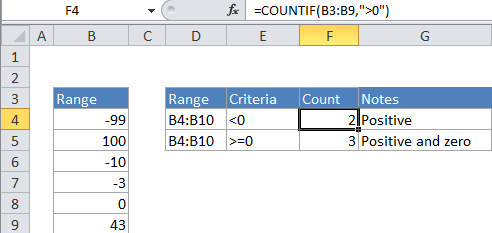## Excel Office

Excel How Tos, Tutorials, Tips & Tricks, Shortcuts

# Count cells that contain positive numbers in Excel

This tutorial shows how to Count cells that contain positive numbers in Excel using the example below;

## Formula

`=COUNTIF(range,">0")`## Explanation

To count positive numbers in a range of cells, you can use the COUNTIF function.

In the example, the active cell contains this formula:

`=COUNTIF(B2:B6,">0")`

### How this formula works

COUNTIF counts the number of cells in a range that match the supplied criteria. In this case, the criteria is supplied as “>0”, which is evaluated as “values greater than zero”. The total count of all cells in the range that meet this criteria is returned by the function.

Also See:   Highlight duplicate values in Excel

You can easily adjust this formula to count cells based on other criteria. For example, to count all cells with a value greater than or equal to 100, use this formula:

`=COUNTIF(range,">=100")`